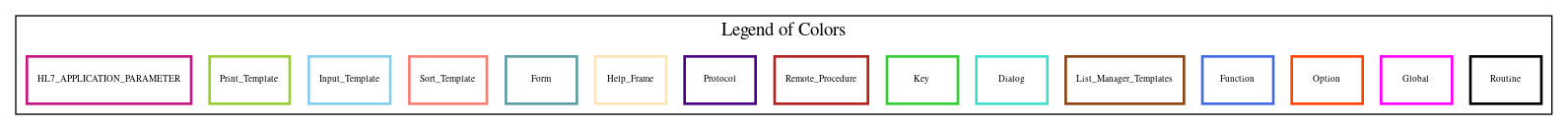Home   Package List   Routine Alphabetical List   Global Alphabetical List   FileMan Files List   FileMan Sub-Files List   Package Component Lists   Package-Namespace Mapping
Routine: MAGDHLTC

# Routine: MAGDHLTC

## Information

MAGDHLTC ;WOIFO/MLH/PMK - IHE-based ADT interface for PACS - trigger events - A11, A12, A13 ;20 Mar 2017 12:15 PM

## Source Information

Source file <MAGDHLTC.m>

## Call Graph### Call Graph Total: 5

Package Total Call Graph
Health Level Seven 2 INIT^HLFNC2  GENERATE^HLMA

## Caller Graph

### Legends:#### Package Component Superscript legend

 action A extended action Ea event driver Ed subscriber Su protocol O limited protocol LP run routine RR broker B edit E server Se print P screenman SM inquire I### Caller Graph Total: 1

Package Total Caller Graph
Imaging 1 MAGDHLT

## Entry Points

A11 ; GOTO entry point from MAGDHLT - patient admission cancel
; INPUT: XDFN IEN of pt whose admission is being cancelled
; XDT date/time of cancellation
; function return: result of message generation from call to GENERATE^HLMA
A12 ; GOTO entry point from MAGDHLT - cancel patient transfer
; INPUT: XDFN IEN of pt whose admission is being cancelled
; XDT date/time of cancellation
; function return: result of message generation from call to GENERATE^HLMA
A13 ; GOTO entry point from MAGDHLT - cancel patient discharge
; INPUT: XDFN IEN of pt whose admission is being cancelled
; XDT date/time of cancellation
; function return: result of message generation from call to GENERATE^HLMA

## External References

Name Field # of Occurrence
INIT^HLFNC2 A11+10, A12+10, A13+10
GENERATE^HLMA A11+30, A12+32, A13+31
\$\$MAKE^MAG7UM A11+26, A12+28, A13+27
LOGGW^MAGDHLL A11+31, A12+33, A13+32
\$\$EVN^MAGDHLS A11+21, A12+21, A13+21
\$\$PID^MAGDHLS A11+22, A12+22, A13+22
\$\$PV1^MAGDHLS A11+23, A12+23, A13+23
\$\$ROL^MAGDHLS A12+24, A13+24

## Local Variables

### Legend:

 >> Not killed explicitly * Changed ! Killed ~ Newed

Name Field # of Occurrence
HL A11+5~, A11+10, A11+11, A12+5~, A12+10, A12+11, A13+5~, A13+10, A13+11
HLA A11+6~, A12+6~, A13+6~
HLA("HLS" A11+26, A11+27!, A12+28, A12+29!, A13+27, A13+28!
>> HLECH A11+16, A12+16, A13+16
>> HLFS A11+15, A12+15, A13+15
MSG A11+8~, A11+21*, A11+22*, A11+23*, A11+26*, A12+8~, A12+21*, A12+22*, A12+23*, A12+24*
A12+25*, A12+28*, A13+8~, A13+21*, A13+22*, A13+23*, A13+24*, A13+27*
MSGA11A A11+7~
MSGA11A(1 A11+14*, A11+15*, A11+16*, A11+17*, A11+18*
MSGA12A A12+7~
MSGA12A(1 A12+14*, A12+15*, A12+16*, A12+17*, A12+18*
MSGA13A A13+7~
MSGA13A(1 A13+14*, A13+15*, A13+16*, A13+17*, A13+18*
>> RESULT A11+30, A11+32, A12+32, A12+34, A13+31, A13+33
U A11+11, A11+32, A12+11, A12+34, A13+11, A13+33
>> XDFN A11+22, A11+23, A12+22, A12+23, A12+24, A12+25, A13+22, A13+23, A13+24
>> XDT A11+21, A11+23, A12+21, A12+23, A13+21, A13+23